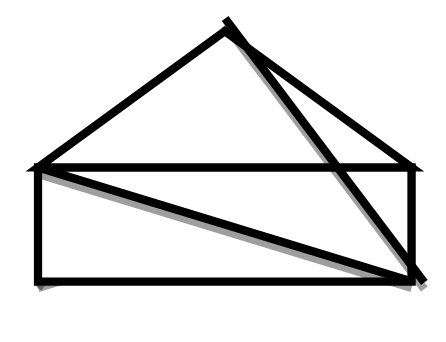EXERCISE 5.8

1) Examine whether the following are polygons, if any one among them is not, say why?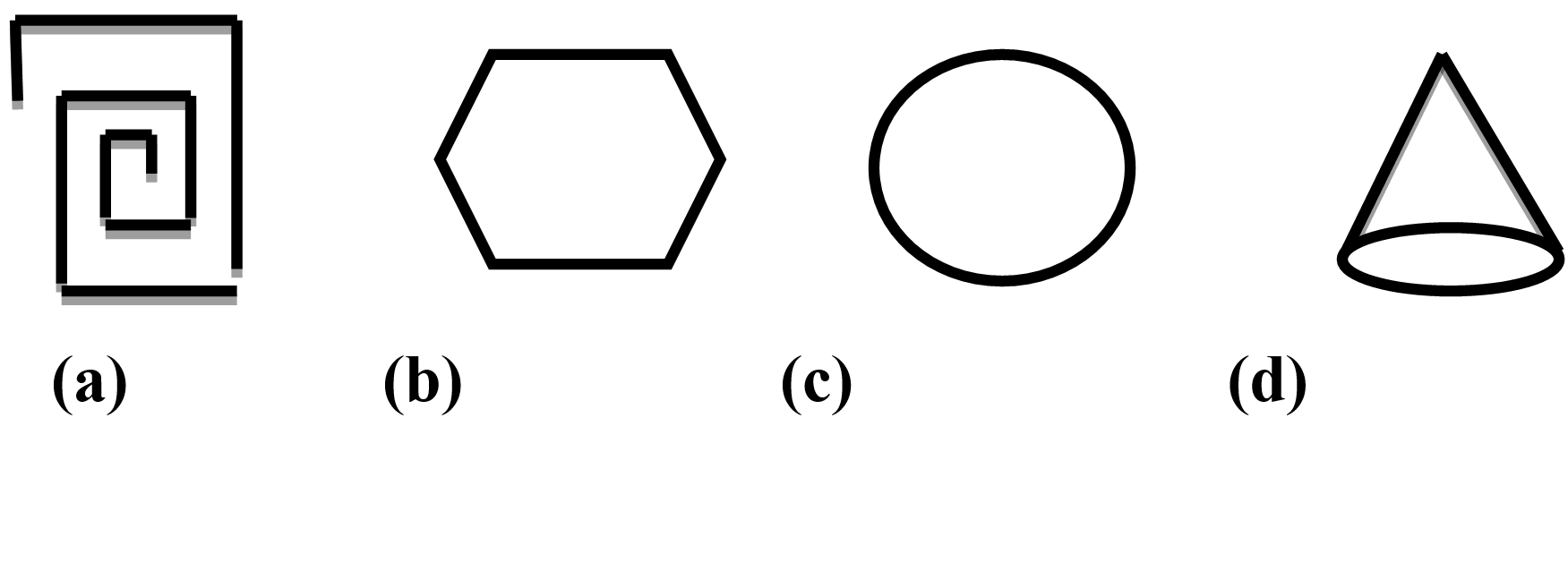(a) Is not a polygon. Since the figure is not closed.
(b) Yes, it is a polygon of six sides.
(c) Not a polygon, as it is not made up of line segments.
(d) Not a polygon, as it is not made up of line segments.

2) Name each polygon :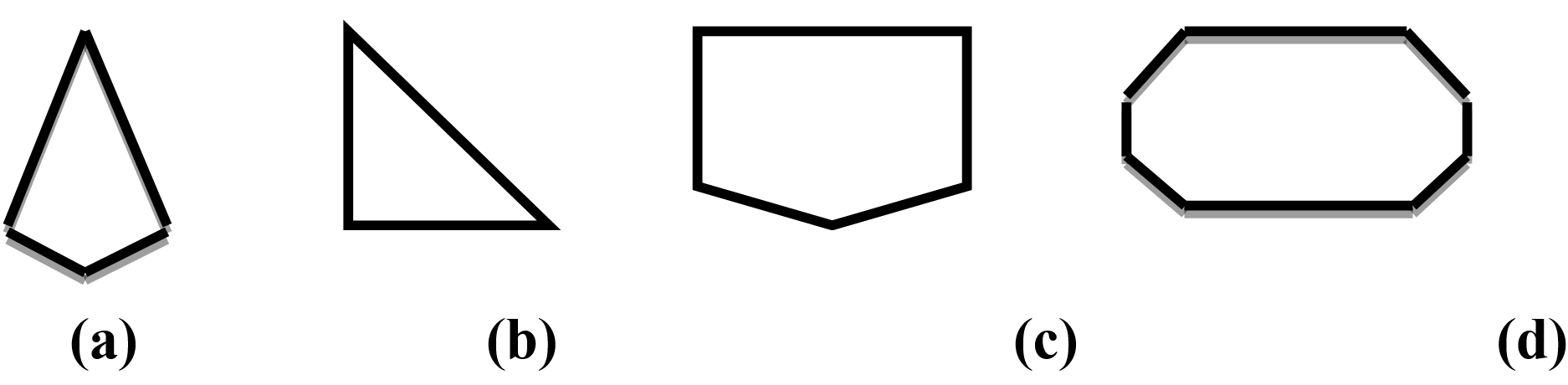Ans : (a) Quadrilateral     (b) Triangle            (c) Pentagon              (d) Octagon

3) Draw a rough sketch of regular hexagon. Connecting any three of its vertices, draw a triangle. Identify the type of the triangle you have drawn.
Ans :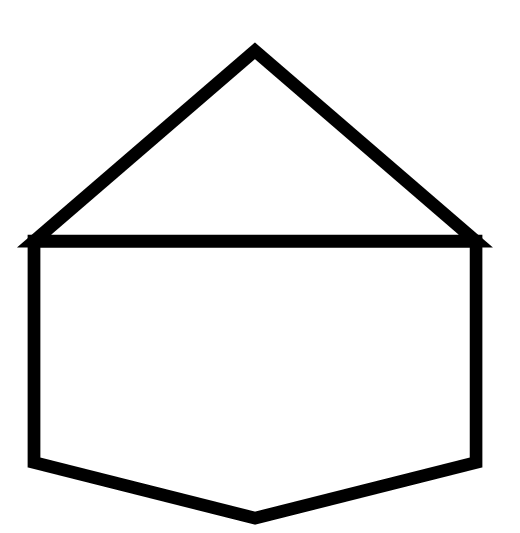We get an equilateral triangle

4) Draw a rough sketch of a regular octagon.(Use squared paper if you wish). Draw a rectangle by joining exactly four of the vertices of the octagon.
Ans :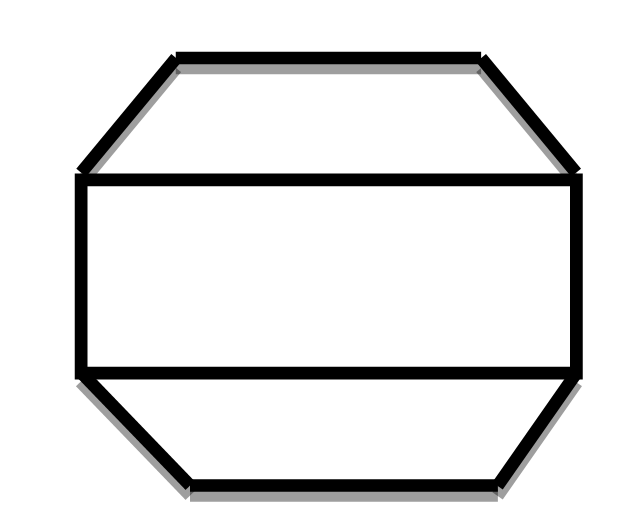5) A diagonal is a line that joins any two vertices of the polygon and is not a side of the polygon.Draw a rough sketch of a pentagon and draw its diagonals.
Ans :